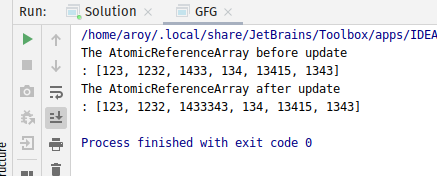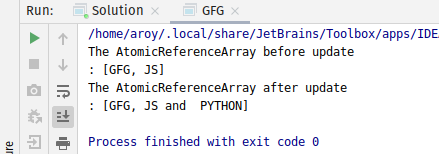# AtomicReferenceArray accumulateAndGet() method in Java with Examples

The accumulateAndGet() method of a AtomicReferenceArray class is used to atomically updates the element at index i of AtomicReferenceArray with the results of applying the given accumulatorFunction to the current and given values and returns the updated value. The accumulatorFunction should be side-effect-free, since it may be re-applied when attempted updates fail due to contention among threads. The function is applied with the current value at index i as its first argument, and the given update as the second argument.

Syntax:

```public final E accumulateAndGet(int i, E x,
BinaryOperator<E> accumulatorFunction)

```

Parameters: This method accepts:

• i which is an index of AtomicReferenceArray to perform the operation accepts,
• x which is the updated value and
• accumulatorFunction which is a side-effect-free function of two arguments.

Return value: This method returns the updated value.

Below programs illustrate the accumulateAndGet() method:
Program 1:

 `// Java program to demonstrate ` `// accumulateAndGet() method ` ` `  `import` `java.util.concurrent.atomic.*; ` `import` `java.util.function.BinaryOperator; ` ` `  `public` `class` `GFG { ` `    ``public` `static` `void` `main(String args[]) ` `    ``{ ` `        ``// an array ` `        ``Integer a[] = { ``123``, ``1232``, ``1433``, ``134``, ``13415``, ``1343` `}; ` ` `  `        ``// AtomicReferenceArray with array ` `        ``AtomicReferenceArray array ` `            ``= ``new` `AtomicReferenceArray<>(a); ` ` `  `        ``// Print AtomicReferenceArray ` `        ``System.out.println( ` `            ``"The AtomicReferenceArray before update\n: "` `            ``+ array); ` ` `  `        ``// Index and Value to apply accumulateAndGet ` `        ``int` `index = ``2``; ` `        ``int` `E = ``343``; ` ` `  `        ``// Declaring the accumulatorFunction ` `        ``// applying function to add value as string ` `        ``BinaryOperator add ` `            ``= (u, v) ` `            ``-> u.toString() ` `                   ``+ v.toString(); ` ` `  `        ``// apply accumulateAndGet() ` `        ``array.accumulateAndGet(index, E, add); ` ` `  `        ``// print AtomicReferenceArray ` `        ``System.out.println( ` `            ``"The AtomicReferenceArray "` `            ``+ ``"after update \n: "` `            ``+ array); ` `    ``} ` `} `

Output:Program 2:

 `// Java program to demonstrate ` `// accumulateAndGet() method ` ` `  `import` `java.util.concurrent.atomic.*; ` `import` `java.util.function.BinaryOperator; ` ` `  `public` `class` `GFG { ` `    ``public` `static` `void` `main(String args[]) ` `    ``{ ` `        ``// an array ` `        ``String a[] = { ``"GFG"``, ``"JS"` `}; ` ` `  `        ``// AtomicReferenceArray with array ` `        ``AtomicReferenceArray arra ` `            ``= ``new` `AtomicReferenceArray<>(a); ` ` `  `        ``// Print AtomicReferenceArray ` `        ``System.out.println( ` `            ``"The AtomicReferenceArray"` `            ``+ ``" before update \n: "` `            ``+ array); ` ` `  `        ``// Index and Value to apply accumulateAndGet ` `        ``int` `index = ``1``; ` `        ``String E = ``" PYTHON"``; ` ` `  `        ``// Declaring the accumulatorFunction ` `        ``// applying function to add value as string ` `        ``BinaryOperator add ` `            ``= (u, v) ` `            ``-> u.toString() ` `                   ``+ ``" and "` `                   ``+ v.toString(); ` ` `  `        ``// apply accumulateAndGet() ` `        ``array.accumulateAndGet(index, E, add); ` ` `  `        ``// print AtomicReferenceArray ` `        ``System.out.println( ` `            ``"The AtomicReferenceArray"` `            ``+ ``" after update \n: "` `            ``+ array); ` `    ``} ` `} `

Output:Attention reader! Don’t stop learning now. Get hold of all the important Java and Collections concepts with the Fundamentals of Java and Java Collections Course at a student-friendly price and become industry ready.

My Personal Notes arrow_drop_upCheck out this Author's contributed articles.

If you like GeeksforGeeks and would like to contribute, you can also write an article using contribute.geeksforgeeks.org or mail your article to contribute@geeksforgeeks.org. See your article appearing on the GeeksforGeeks main page and help other Geeks.

Please Improve this article if you find anything incorrect by clicking on the "Improve Article" button below.

Article Tags :
Practice Tags :

Be the First to upvote.

Please write to us at contribute@geeksforgeeks.org to report any issue with the above content.